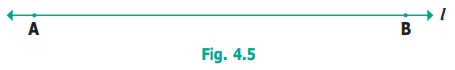Home | | Maths 6th Std | Describing lines

# Describing lines

A line can be long or short. A line can be flat or slant or vertical. If a line is rotated in any direction, it remains to be a line.

Describing lines

A line can be long or short. A line can be flat or slant or vertical. If a line is rotated in any direction, it remains to be a line. So, given below (Fig. 4.3) are lines in different positions.But the following (Fig.4.4) are not lines.If the length of a line is ignored, then it can be extended in both the directions without ending as in (Fig. 4.5) given below. A line through two points A and B is written asor. Also it is denoted by a letter ' l ' .Tags : Geometry | Term 1 Chapter 4 | 6th Maths , 6th Maths : Term 1 Unit 4 : Geometry
Study Material, Lecturing Notes, Assignment, Reference, Wiki description explanation, brief detail
6th Maths : Term 1 Unit 4 : Geometry : Describing lines | Geometry | Term 1 Chapter 4 | 6th Maths